Reach Us+44-1904-929220
Existence and Stability of Triangular Points in the Relativistic R3bp When the Primaries are Triaxial Rigid Bodies and Sources of Radiation | OMICS International
Journal of Astrophysics & Aerospace Technology
All submissions of the EM system will be redirected to Online Manuscript Submission System. Authors are requested to submit articles directly to Online Manuscript Submission System of respective journal.

# Existence and Stability of Triangular Points in the Relativistic R3bp When the Primaries are Triaxial Rigid Bodies and Sources of Radiation

 Jagadish Singh1 and Nakone Bello2* 1Department of Mathematics, Faculty of Science, Ahmadu Bello University, Zaria, Nigeria 2Department of Mathematics, Faculty of Science, Usmanu Danfodiyo University, Sokoto, Nigeria *Corresponding Author : Nakone Bello Department of Mathematics Faculty of Science Usmanu Danfodiyo University, Sokoto, Nigeria E-mail: [email protected] Received: April 01, 2016 Accepted: April 14, 2015 Published: April 18, 2016 Citation: Singh J, Bello N (2016) Existence and Stability of Triangular Points in the Relativistic R3bp When the Primaries are Triaxial Rigid Bodies and Sources of Radiation. J Astrophys Aerospace Technol 4:131. doi:10.4172/2329-6542.1000131 Copyright: © 2016 Singh J, et al. This is an open-access article distributed under the terms of the Creative Commons Attribution License, which permits unrestricted use, distribution, and reproduction in any medium, provided the original author and source are credited.

Visit for more related articles at Journal of Astrophysics & Aerospace Technology

#### Abstract

This paper deals with the triangular points and their linear stability in the relativistic R3BP when the primaries are triaxial rigid bodies and sources of radiation. It is observed that the locations of the triangular points are affected by the relativistic terms, radiation pressure forces and the triaxiality of the primaries. It is also seen that for these points the range of stability region increases or decreases according as p >0 or p<0 where p depends upon the relativistic terms, the radiation and triaxiality coefficients.

 Keywords Celestial mechanics; Radiation; Triaxiality; Relativity; R3BP Introduction The restricted three-body problem possesses five stationary solutions called Lagrangian points, three of which called collinear equilibria lie on the line joining the primaries and the other two called equilateral equilibria make equilateral triangles with primaries. In general, the collinear equilibria are unstable while equilateral ones are stable, in the Lyapunov sense, only in a certain region for the mass parameter. Various authors have made studies on Lagrangian points in the restricted three-body problem by considering the more massive primary or both primaries as source of radiation. Some of the important contributions are by Radzievskii [1,2] Simmons et al.  Kunitsyn and Tureshbaev  Singh and Ishwar  Singh . Some of the significant studies, by considering the triaxiality of one or both primaries, are Khanna and Bhatnagar  and Singh . Sharma et al. [9,10] have studied the stationary solutions of the planar restricted three-body problem when one or both primaries are sources of radiation and triaxial rigid bodies with one of the axes as the axis of symmetry and the equatorial plane coinciding with the plane of motion. The theory of the general relativity is currently the most successful gravitational theory describing the nature of space and time and well confirmed by observations . Brumberg [12,13] studied the problem in more details and collected most of the important results on relativistic celestial mechanics. He did not obtain only the equations of motion for the general problem of the three bodies but also deduced the equation of the motion for the restricted problem of three bodies. Bhatnagar and Hallan  investigated the existence and linear stability of the triangular point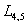in the relativistic R3BP. They concluded thatare always unstable in the whole range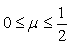in contrast to the classical R3BP where they are stable for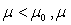being the mass ratio and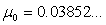is the Routh’s value. Douskos and Perdios  investigated the stability of the triangular points in the relativistic R3BP and contrary to the results of Bhatnagar and Hallan , they obtained a region of linear stability in the parameter space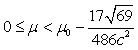whereis Routh’s value. Katour et al.  obtained new locations of the triangular points in the framework of relativistic R3BP with oblateness and photogravitional corrections to triangular points. Singh and Bello [17,18] studied the motion of a test particle in the vicinity of the triangular pointsby considering one or both primaries as the sources of radiation in the framework of the relativistic restricted three-body problem (R3BP). In the present work, we study the existence of the triangular points and their linear stability by considering both primaries as triaxial rigid bodies and sources of radiation. This paper is organized as follows: In Sect. 2, the equations governing the motion are presented; Sect. 3 describes the positions of triangular points, while their linear stability is analyzed in Sect.4; The discussion of the results is given in Sect. 5. In Sect. 6, we conclude our work and highlight the differences and similarities between our work and previous works. Equations of Motion In our recent papers Singh and Bello [17,18] we have studied the effect of radiation pressure of the primaries in the relativistic R3BP and found that the positions of triangular points and their stability are affected by both relativistic and radiation factors. In this paper we extend this work by considering both primaries as triaxial rigid bodies as well as sources of radiation. The pertinent equations of motion of an infinitesimal mass in the relativistic R3BP in a barycentric synodic coordinate system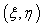and dimensionless variables can be written as Singh and Bello [17,18]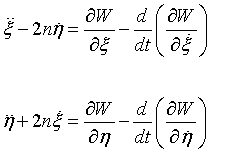(1) with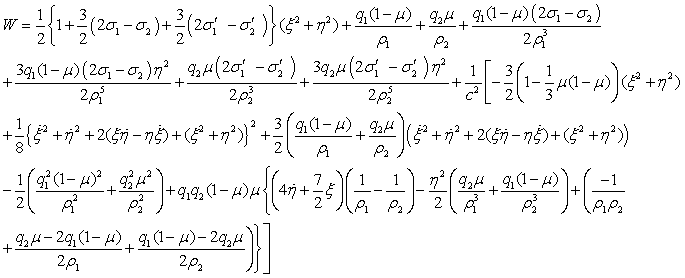(2)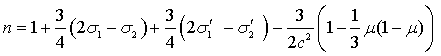(3)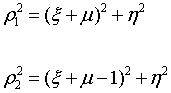(4) where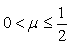is the ratio of the mass of the smaller primary to the total mass of the primaries,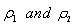are distances of the infinitesimal mass from the bigger and smaller primary, respectively; n is the mean motion of the primaries; cis the velocity of light.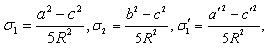and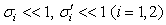characterize the triaxiality of the bigger and smaller primary with a, b, c as lengths of the semi-axes of the bigger primary and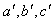′ as those of the smaller primary. The radiation factor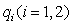is given by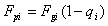such that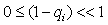Radzievskii  where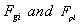are respectively the gravitational and radiation pressure. Here as Katour et al.  we do not include the parameters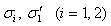in the relativistic part of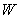since the magnitude of these terms is so small due to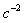where c is the speed of light. Location of Triangular Points The libration points are obtained from equation (1) after putting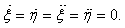These points are the solutions of the equations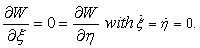That is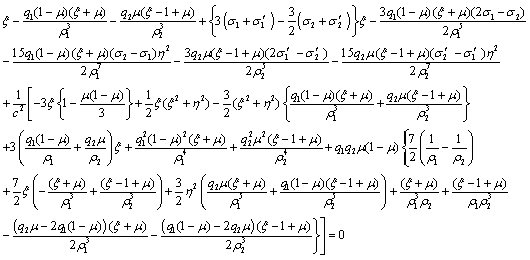(5) and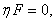with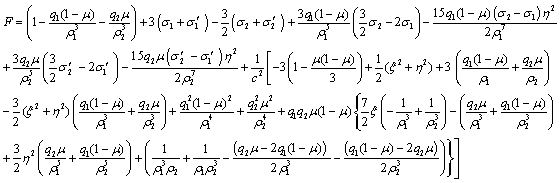For simplicity, putting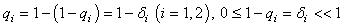and neglecting second and higher order terms in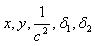c and their products. Following as our papers [17,18] we have obtained from the system (5) with η ≠ 0, the coordinates of the triangular points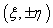as: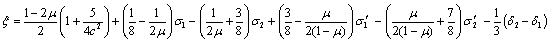(6) Stability of L4,5 Since the nature of the linear stability about the point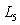will be similar to that about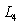, it will be sufficient to consider here the stability only near. Let (a,b) be the coordinates of the triangular pointWe set, in the equations (1) of motion. First, we compute the terms of their R.H.S, neglecting second and higher order terms, we get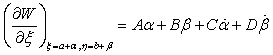where,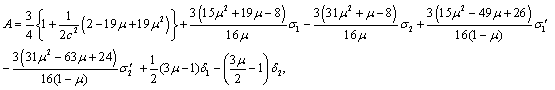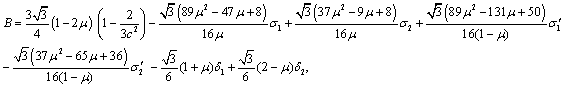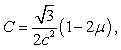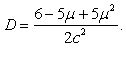Similarly, we obtain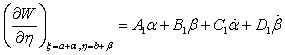where,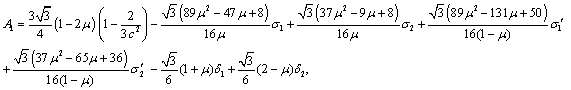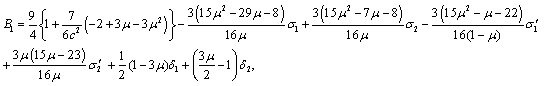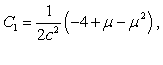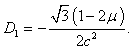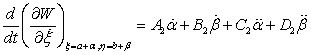where,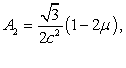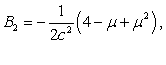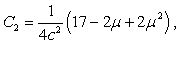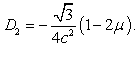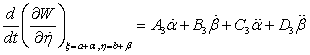where,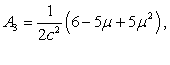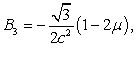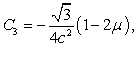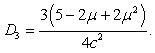The characteristic equation of the variational equations of motion corresponding to (1) can be expressed as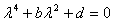(7) where,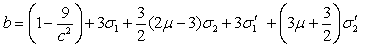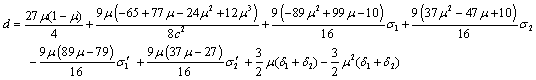For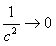when the primaries are non-luminous and spherical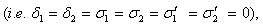Eq. (7) reduces to its well-known classical restricted problem form (See e.g. Szebehely ).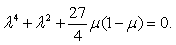The discriminant of (7) is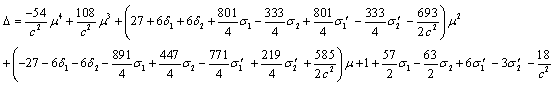(8) Its roots are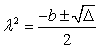(9) where,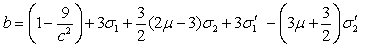From (8), we have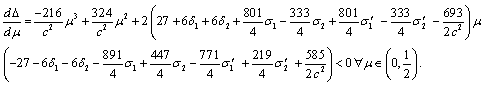(10) From (10), it can be easily seen that Δ is monotone decreasing in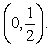But(11)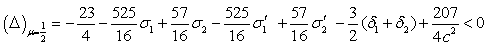Since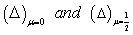are of opposite signs, andΔ is monotone decreasing and continuous, there is one value of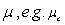in the interval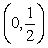for which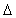vanishes. Solving the equation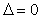using (8), we obtain the critical value of the mass parameter as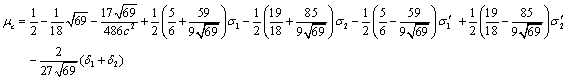(12)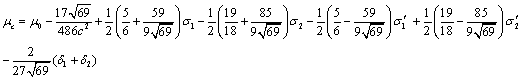whereis the Routh’s value. We consider the following three regions of the values of μ separately. When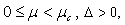the values of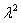given by (9) are negative and therefore all the four characteristic roots are distinct pure imaginary numbers. Hence, the triangular points are stable. When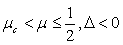the real parts of the characteristic roots are positive. Therefore, the triangular points are unstable. When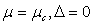the values ofgiven by (9) are the same. This induces instability of the triangular points. Hence, the stability region is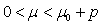(13) with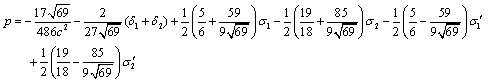Discussion In this section, we discuss the triangular libration points in the relativistic restricted three-body problem, under the assumption that the primaries are luminous and triaxial. The positions of the triangular points in equation (6) are obtained. It can be seen that they are affected by the relativistic, radiation and triaxiality factors. It is important to note that these triangular libration points cease to be classical one i.e., they no longer form equilateral triangles with the primaries. Rather they form scalene triangles with the primaries. Equation (12) gives the critical value of the mass parameter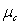of the system which depends upon relativistic factor, triaxiality parameters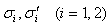and radiation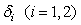. In the absence of relativistic factor, the results obtained in this study are in agreement with those of Sharma et al.  Singh  when there is no perturbations in the Coriolis and centrifugal forces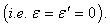When the primaries are non triaxial, the results of the present study tally with those of Singh and Bello . It is noticeable from (13) that that radiation and triaxiality all have destabilizing effects, and therefore the size of the range of stability decreases with increase of the values of these parameters. Evidently, it can also seen that the relativistic factor reduces the size of stability region. When the primaries are non-luminous and non-triaxial, the stability results obtained in this study are in accordance with those of Douskos and Perdios  and disagree with Bhatnagar and Hallan . In the absence of relativistic factor, the results obtained in this study are in agreement with those of Sharma  and those of Singh  when the perturbations are absent. When the primaries are oblate spheroids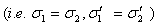, the results of equation (6) in this study differ from those of katour et al.  when the radiation terms are absent in the realistic part of the potential W Conclusion By considering the primaries as triaxial rigid bodies and sources of radiation in the relativistic CR3BP, we have determined the positions of the triangular points and their linear stability. It is found that their positions and stability region are affected by relativistic, triaxiality and radiation factors. It is further observed that the relativistic, triaxiality and radiation factors have destabilizing tendencies resulting in a decrease in the size of the region of stability. We have noticed that the expressions for A, D, A2, C2 in Bhatnagar and Hallan  differ from the present study when the radiation pressure factors are absent and the primaries are spherical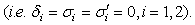Consequently, the characteristic equation is also different. This led them Bhatnagar and Hallan  to infer that the triangular points are unstable, contrary to Douskos and Perdios and our results. Our results are also in disagreement with those of Katour et al. . One major distinction is that the expression of the mean motion which they used in their study differ from our own. It seems that there is an error in their expression. References Radzievskii VV (1950) The restricted problem of three bodies taking account of light pressure, Astron. Z 27: 246-250. Radzievskii VV (1953) The space photogravitational restricted three-body problem. Astron. Z 30: 256-273. Simmons JFL, Mc Donald AJC, Brown JC (1985) The restricted 3-body problem with radiation pressure. CelestMech 35: 145-187. Kunitsyn AL, Turesbaev AT (1985) Stability of the coplanar libration points in the photogravitational restricted three-body problem. Soviet Astron. 11: 391-392. Singh J, Ishwar B (1999) Stability of triangular points in the generalized photogravitational restricted three-body problem. Bull AstrSoc India 27: 415-424. Singh J (2009) Combined effects of oblateness and radiation on the nonlinear- stability of L4 in the restricted three-body problem. Astron J 137: 3286-3292. Khanna M, Bhatnagar KB (1999) Existence and stability of libration points in the restricted three-body problem when the smaller primary is a triaxial rigid body and the bigger one an oblate spheroid. Indian J. pure appl. Math 30: 721-733. Singh J (2013) The equilibrium points in the perturbed R3BP with triaxial and luminous primaries. Astrophys Space Sci 1: 41-50. Sharma RK, Taqvi ZA, Bhatnagar KB (2001) Existence and stability of the libration points in the restricted three-body problem when the bigger primary is a triaxial rigid body and source of radiation. Indian J. Pure Appl Math 2: 255-266. Sharma RK, Taqvi ZA, Bhatnagar KB (2001) Existence and stability of the libration points in the restricted three-body problem when the primaries are triaxial rigid bodies and sources of radiation. Indian J Pure Appl Math 7: 981-994. Will CM (2014) The confrontation between general relativity and experiment. Living Rev Relativity 17: 4. Brumberg VA (1991) Essential Relativistic Celestial Mechanics. Adam Hilger, New York. Brumberg VA (1972) Relativistic Celestial Mechanics. Nauka, Moscow. Bhatnagar KB, Hallan PP (1998) Existence and stability of L4,5  in the relativistic restricted three-body problem. Celest. Mech. Dyn. Astron 69: 271-281. Douskos CN, Perdios EA (2002) “On the stability of equilibrium points in the relativistic restricted three-body problem”. Celest. Mech. Dyn. Astron 82: 317-321. Katour DA, Abd El-Salam FA, Shaker MO (2014) Relativistic restricted three-body problem with oblateness and photo-gravitational corrections to triangular equilibrium points. Astrophys Space Sci 351: 143-149. Singh J, Bello N (2014) Effect of radiation pressure on the stability of L4,5 in relativistic R3BP. Astrophys Space Sci 2: 483-490. Singh J, Bello N (2014) On the stability of L4,5 in the photogravitational relativistic R3BP. DifferEquDyn Syst. Cuskey MSW (1963) Introduction to celestial mechanics, Addision-Wesley. Szebehely V (1967) Theory of orbits. The restricted problem of three bodies. Academic Press, New York, USA.
Select your language of interest to view the total content in your interested language

### Article Usage

• Total views: 9051
• [From(publication date):
December-2016 - Nov 12, 2019]
• Breakdown by view type
• HTML page views : 8917Can't read the image? click here to refresh